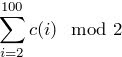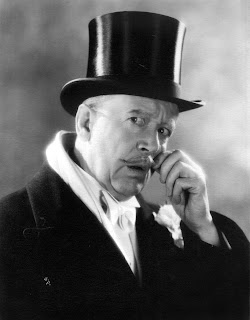## Archive for the puzzles Category

Posted in puzzles on August 12, 2008 by Philonous

Ok, so here’s the long awaited solution.

Let us suppose for a moment that in fact all the beads are of the same colour, i.e. indistinguishable. Then we know that they will return to the same configuration: any collision of two beads may as well be thought of as beads passing straight through each other so we will have the same configuration after one second.

Now to the problem. Here we can distinguish between beads. Nonetheless, by the previous argument, we know that after a second, the configuration of the beads will be the same apart from some of the beads perhaps being swapped. Well performing this permutation of beads again and again must lead to the original position at some point. Et voila.

Posted in puzzles on August 5, 2008 by Philonous

Here’s a great puzzle I heard at a conference in Leicester the other week.

On a circle of wire are a number of (point) beads moving at the same constant speed; for simplicity’s sake let’s suppose that they would complete one complete circuit in a second. Since they are not all necessarily moving in the same direction, they may collide – we assume that any such collision is completely elastic, that is, they are still moving at the same speed after the collision, just in opposite directions. Suppose all the beads are different colours.

Given any starting position for the beads, will they return to precisely this position in some finite time?

## 100 hat solution

Posted in maths, puzzles with tags on April 16, 2008 by Philonous
I suddenly realised that I haven’t yet posted the solution to the hundred hat problem.

In slightly mathematical language, here’s the solution. Replace black by 0 and white by 1. Let the ith prisoner have hat colour c(i). The first person shouts out the value ofThe next person does the calculation
and shouts his answer out. In general, since the kth prisoner knows c(2), . . . , c(k-1), (having heard them shouted), c(k+1), . . . , c(100) (being able to see them) andcourtesy of the first prisoner’s shout, he can calculate c(k) = (c(k) mod 2). Of course, here we’ve set the number of prisoners to 100, but this isn’t necessary.For non-mathematically literate folk:

The idea really isn’t complicated at all – if it seems so, then I’ve explained it badly. First of all, knowing if a hat is white or not is equivalent to knowing its colour since there are only two. The first person to go counts up the number of white hats he can see in front of him. If it’s odd, he shouts ‘white’ and if it’s even, he shouts ‘black’. Prisoner number 2 then counts the number of white hats in front of him. He then figures out if it’s odd or even. We know that

• (odd number) – (odd number) = even number
• (odd number) – (even number) = odd number
• (even number) – (odd number) = odd number
• (even number) – (even number) = even number

Prisoner 2 can use prisoner 1’s shout as the first number and his own tally as the second. This spits out something on the right hand side. If he gets ‘even’ he shouts black, if he gets ‘odd’ he shouts white. Prisoner 3 has heard prisoner 1 and 2 and also knows whether the number of hats in front is even or odd. He then does a similar calculation and shouts out the right answer…and so on.

Perhaps this isn’t perfectly explained. Never mind.

## 100 Lamp solution, 100 Hat problem.

Posted in maths, puzzles with tags on April 12, 2008 by Philonous

Yesterday, I posted a little puzzle about a hundred blind lamp lighters. Don’t read on if you’d like to give this a go for yourself.

Here’s the solution: The squared number lamps will be on at the end, i.e. 1,4,9,16,25,36,49…etc.

We need to find the number of lamps which are on at the end. So let’s try and figure out in what circumstances a given lamp will be on. As an example, think about whether the 6th lamp will be on. It’s turned on by the 1st guy, off by the 2nd, on by the 3rd, and then off by the 6th. Notice that we’ve just listed all of the numbers that divide 6, i.e. 1,2,3,6. In general, for a lamp to be off, the button should have been pressed an even number of times; for it to be on, the button should have been pressed an odd number of times. For the kth lamp, the number of times it is pressed is the number of numbers dividing k.

Now let’s look at another example. The 12th lamp will be pressed by guys number 1, 2, 3, 4, 6, 12. We can split these divisors into pairs: (1,12), (2,6), (3,4) where multiplying the numbers together gives 12. Since we can pair these things up, there is an even number of divisors.

Suppose now for example, that we take the number 36. Here we have divisors 1, 2, 3, 4, 6, 9, 12, 18, 36. In this case, we have the pairs (1,36), (2,18), (3,12), (4,9), and 6 is paired with itself. Here we have an odd number of divisors, the reason being that one number (6) is paired with itself. So a general number k has an odd number of divisors if (and only if) one divisor is paired with itself, that is, there is some number which multiplied by itself gives k. In less obtruse words, k is a square number.

And there’s the proof. (Note that actually, the number of lamps doesn’t matter as long as it’s the same as the number of lamp lighters.)

Last night after the Pure Postgraduate seminar, a post-pub discussion brought up the following hundred hat problem:

Here’s another problem, this time with a hundred hats. Suppose that there is a strange kingdom where logical prowess is prized above all other things. 100 prisoners languish in prison all sentenced to death. Since the king is a nice sort of fellow, he decides that he will set the following challenge: The prisoners are all lined up facing along the line so that a given prisoner can see all the people infront of him, but not himself or the people behind him. A hat is put on each prisoner, either white or black. The prisoner at the back (who can see everyone else’s hat but not his own) then shouts out the colour he thinks his hat is. If he gets the answer right, he is allowed to live. If he gets the answer wrong, he dies. The same process is repeated by the prisoner second from the back and so on.

The prisoners are allowed to confer before the whole process and so can decide on a strategy. How many prisoners can defintitely be saved by an appropriate strategy?(Hint: At least half can be saved. The guy at the back can’t be helped: he may as well shout randomly. He could however, help the guy in front of him. If they decide beforehand that the first guy will shout the colour of the hat in front of him, then at least he can save the second guy who will then know his own hat colour. Continuing the process, we can save for certain at least every other man. In fact, they can do a lot better…)

## Too many Lamp Lighters

Posted in maths, puzzles with tags on April 11, 2008 by Philonous

Yesterday, as on every Thursday, Manchester University’s geometers, mathematical physicists and topologists gather for the Geometry Seminar. The talk itself was a really great proof of the Mischenko-Fomenko conjecture about the existence maximal complete commutative subalgebras of finite dimensional Lie algebras. Alexei Bolsinov gave a geometric proof of one of the subcases of the proof using some quite neat parts of the theory.

As per usual, we decamped to the pub afterward to discuss anything and everything. Soon enough, one of my supervisors started posing interesting little mathematical problems. Here is one which “of course, the school children know how to solve” which occupied a some minutes in an otherwise boring sleepless night.
Suppose there are a hundred push-button lamps and a hundred blind lamplighters. All lamps are initially off. The first lamplighter passes along the row and pushes each button in turn thereby turning every lamp on. The second lamplighter passes along the row afterward and pushes every other button regardless of whether the lamp is on or off. (So now all the even numbered lamps are off.) The third lamplighter passes along the row and pushes every third button, the fourth pushes every fourth button and so on. After all of the hundred lamplighters have passed by, which of the lamps remain lit?For the answer and an explanation, see tomorrow.